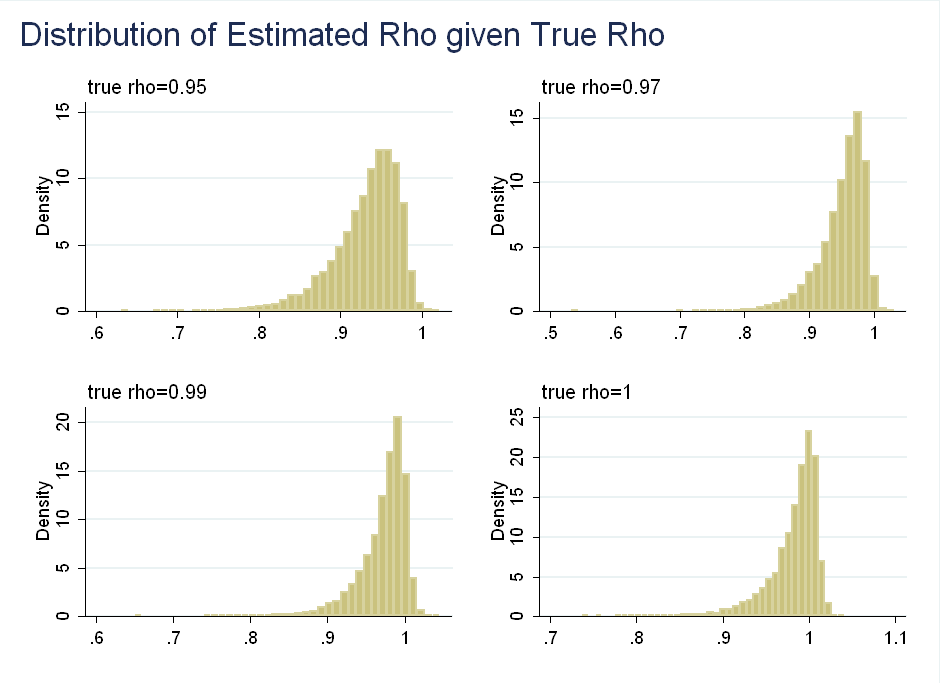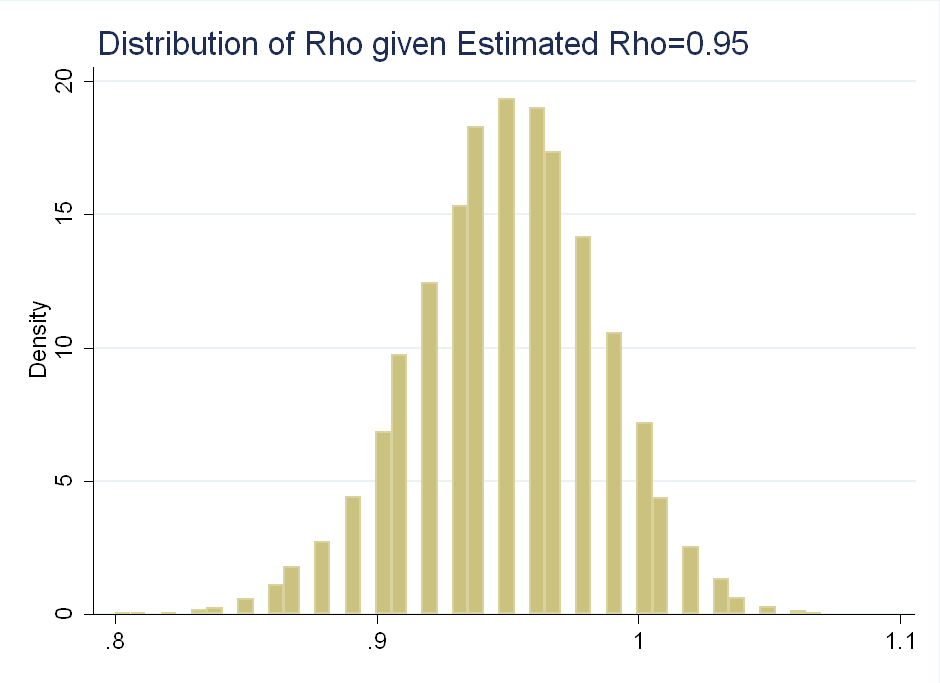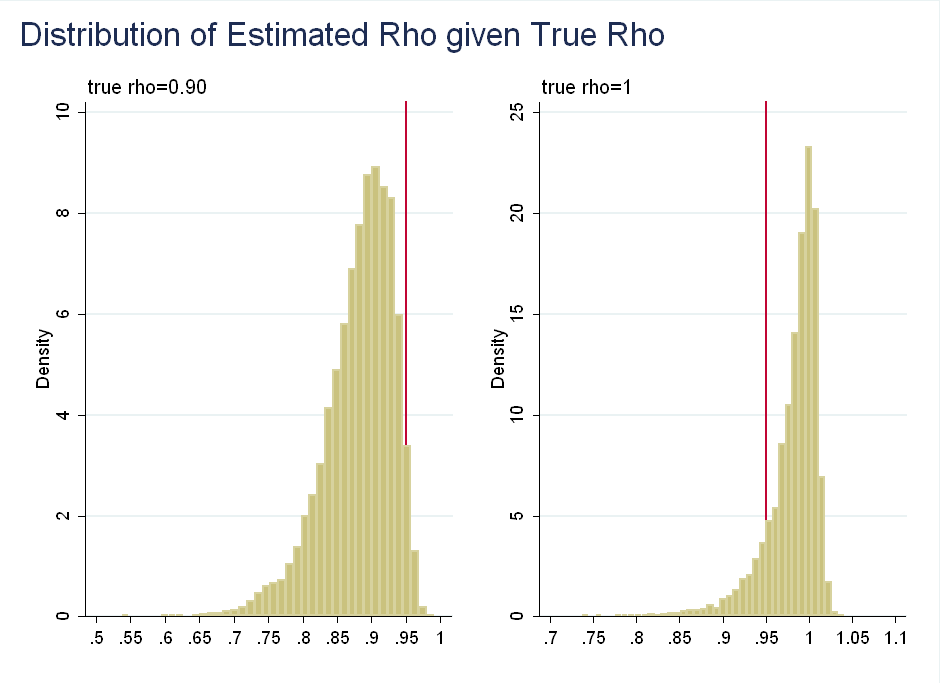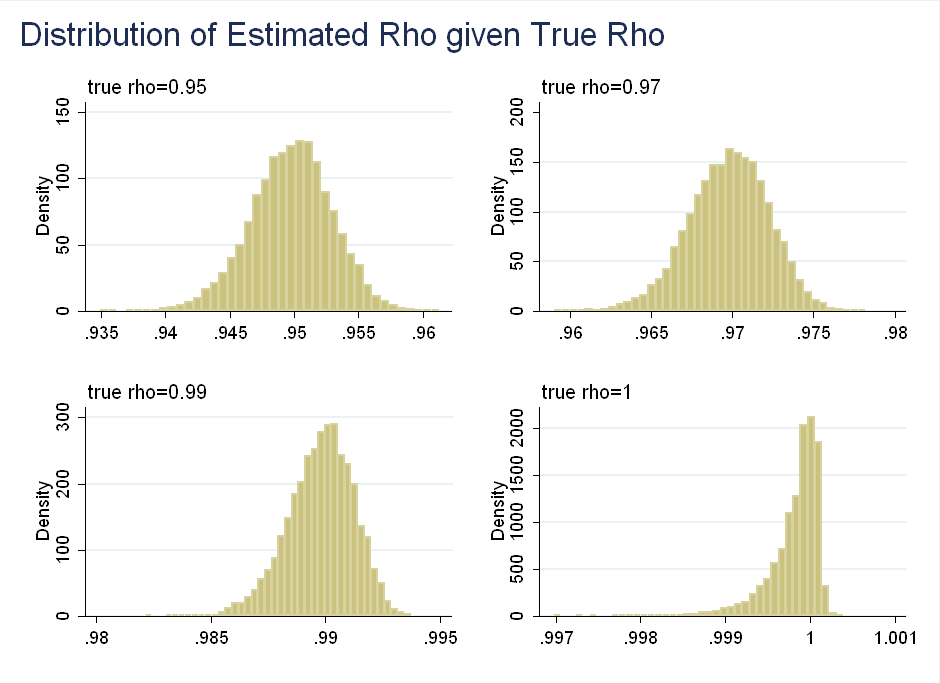Sims and Uhlig argue: although classical (frequentist) $$p$$-values are asymptotically equivalent to Bayesian posteriors, they should not be interpreted as probabilities. This is because the equivalence breaks down in non-stationary models.
The paper uses small sample sizes, with $$T=100$$ - This post examines how the results change with $$T=10,000$$, when the asymptotic behavior kicks in.

# The Setup

Consider a simpler AR(1) model: \begin{equation} y_t=\rho y_{t-1} + \epsilon_t \end{equation} To simplify things, suppose $$\epsilon_t \sim N(0,1)$$. Classical inference suggests that for $$|\rho|<1$$, the estimator is asymptotically normal and converges at rate $$\sqrt{T}$$: \begin{equation} \sqrt{T}(\hat{\rho}-\rho) \rightarrow^{L} N(0,(1-\rho^2)) \end{equation} For $$\rho=1$$, however, we get a totally different distribution, which converges at rate $$T$$, instead of rate $$\sqrt{T}$$: \begin{equation} T(\hat{\rho}-\rho)=T(\hat{\rho}-1)= \rightarrow^{L} \frac{(1/2)([W(1)]^2-1)}{\int_0^1 [W(r)]^2 dr} \end{equation} where $$W(1)$$ is a Brownian motion. Although it looks complicated, it is easier to visualize when you see $$W(1)^2$$ is actually a $$\chi^2(1)$$ variable. This is left skewed, as the probability that a $$\chi^2(1)$$ is less than one is 0.68 and large realizations of $$[W(1)]^2$$ in the numerator get down-weighted by a large denominator (it is the same Brownian motion in the numerator and denominator). In the paper, the authors choose 31 values of $$\rho$$ between 0.8 to 1.1 in increments of 0.01. For each $$\rho$$ they simulate 10,000 samples of the AR(1) model described above with $$T=100$$. Finally, they run an OLS regression of $$y_t$$ on $$y_{t-1}$$ to get the distributions for $$\hat{\rho}$$ (the OLS estimator of $$\rho$$). Below I show the distribution of $$\hat{\rho}$$ for selected values of $$\rho$$:Another way to think about the data is to look at the distribution of $$\rho$$ given observed values of $$\hat{\rho}$$. This is symmetric about 0.95:Their problem with using $$p$$-values as probabilities is that if we observe $$\hat{\rho}=0.95$$, we can reject the null of $$\rho=0.9$$, but we fail to reject the null of $$\rho=1$$ (think about the area in the tails after normalizing the distribution to integrate to 1), even though $$\rho$$ given $$\hat{\rho}$$ is roughly symmetric about 0.95:The problem is distortion by irrelevant information: Values of $$\hat{\rho}$$ much below 0.95 are more likely given $$\rho=1$$ than are values of $$\hat{\rho}$$ much above 0.95 given $$\rho=0.9$$. This is irrelevant as we have already observed $$\hat{\rho}=0.95$$, so we know it is not far above or below.

The prior required to generate these results (i.e. the prior that would let us interpret $$p$$-values as posterior probabilities) is sample dependent. Usually, classical inference is asymptotically equivalent to Bayesian inference using a flat prior, but it is not the case here. The authors show that classical analysis is implicitly putting progressively more weight on values of $$\rho$$ above one as $$\hat{\rho}$$ gets closer to 1.

# Testing with Larger Samples

At first, I found the results counter-intuitive. The first figure above shows that the skewness arrives gradually in finite samples. This is strange, because the asymptotic properties of $$\hat{\rho}$$ are only non-normal for $$\rho=1$$. I figured this was the result of using small samples of $$T=100$$. Under a flat prior, the distribution of $$\rho$$ given the data and $$\epsilon_t$$ having variance of 1 is: \begin{equation} \rho \sim N(\hat{\rho}, (\sum\limits_{t=1}^T y_{t-1})^{-1}) \end{equation} This motivates my intuition for why the skewness arrives slowly: even for small samples, as $$\rho$$ gets close to 1, $$\sum\limits_{t=1}^T y_{t-1}$$ can be very large.

I repeat their analysis, except instead of $$T=100$$, I use $$T=10,000$$. As you can see, the asymptotic behavior kicks in and the skewness arrives only at $$\rho=1$$:I also found that for $$T=10,000$$ the distribution of $$\rho$$ conditional on $$\hat{\rho}$$, does not spread out more for smaller values of $$\hat{\rho}$$, that is a small sample result.

# Conclusion

The point of this paper is to show that the classical way of dealing with unit roots implicitly makes undesirable assumptions - you need a sample-dependent prior which puts more weight on high values of $$\rho$$. To a degree, the authors’ results are driven by the short length of the simulated series. The example where you reject $$\rho=0.9$$ but fail to reject $$\rho=1$$ wouldn’t happen in large samples, as the asymptotic kick in and faster rate of convergence for $$\rho=1$$ gives the distribution less spread.

For now, however, the authors’ criticism is still valid. With quarterly data from 1950-Present you get about 260 observations. Macroeconomics will have to survive until year 4,450 for there to be 10,000 observations, and that’s a long way off.